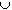#Interactive Real Analysis

Next | Previous | Glossary | Map

## 7.3. Measures

### Examples 7.3.10(b): Properties of Measure

What is the measure of the set Q of all rational numbers and the set I of all irrational numbers inside [0, 1].
We have already shown that a countable set has outer measure zero, and that sets with outer measure zero are measurable. Therefore Q is measurable with m(Q) = 0.

The set [0, 1] is an interval, hence it is measurable with m([0, 1]) = 1 - 0 = 1. Also, the set I = [0, 1] - Q, so that I is also measurable. Since Q and I are disjoint, we can use additivity of measure:

1 = m( [0, 1] ) = m(QI) = = m(Q) + m(I) = 0 + m(I)
Therefore m(I) = 1.
Next | Previous | Glossary | Map